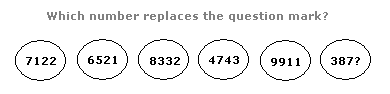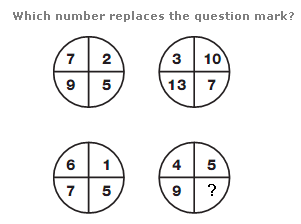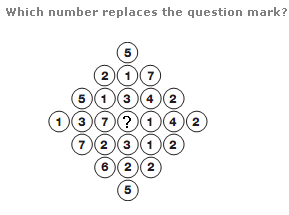# Puzzles - Number puzzles

### Exercise :: Number puzzlesAnswer : 4 Explanation : Adding up the digits in each oval shape, as you move down the column this total increases by 2 each time, from 12 to 22.Answer : 1 Explanation : In each circle, the number in the lower left segment equals the sum of the numbers in the top two segments, and the number in the lower right circle equals the difference between the numbers in the top two segments.Answer : 2 Explanation : Working in rows, the sum of numbers in each row follows the sequence 5, 10, 15, 20, 15, 10, 5.